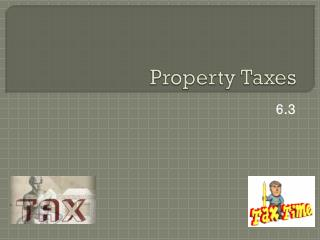# Property Taxes - PowerPoint PPT PresentationDownload PresentationProperty Taxes

Property TaxesDownload Presentation## Property Taxes

- - - - - - - - - - - - - - - - - - - - - - - - - - - E N D - - - - - - - - - - - - - - - - - - - - - - - - - - -
##### Presentation Transcript

1. Decimal Tax Rate • Terms to Know: • Property Tax • Assessed Value • Decimal Tax Rate • Decimal Tax Rate formula= Amount to be Raised by Property tax Total Assessed Value

2. Decimal Tax Rate (Example) Paynesville School District budgeted expenses of \$6,000,000. Estimated income of \$1,800,000. Total assessed value last year was \$39,000,000. Find the tax rate needed to meet expenses, correct to five decimal places.

3. Decimal Tax Rate (Solution) Expenses – Estimated income = Property tax needed \$6,000,000 - \$1,800,000 = \$4,200,000. Solve the Decimal Tax Rate formula… \$4,200,000 / 39,000,000 = 0.107692 = 0.10769 (Tax rate)

4. Check for Understanding (Solution A) • What is the decimal tax rate (3 decimal places)? • Expenses – Estimated income = Property tax needed \$6,750,000 - \$650,000 = \$6,100,000. • Solve the Decimal Tax Rate formula… \$6,100,000 / 80,000,000 = 0.0762 = 0.076 (Tax rate)

5. Check for Understanding (Solution B) • What is the decimal tax rate (4 decimal places)? • Expenses – Estimated income = Property tax needed =\$1,950,000. • Solve the Decimal Tax Rate formula… \$1,950,000 / 48,200,000 = 0.04045 = 0.0405 (Tax rate)

6. Tax Rates per \$100 of Assessed Value (Example) • Calculate the property tax due on Watson’s \$60,000 property if his tax rate is \$6.20 per \$100. • Divide assessed value by 100 \$60,000/100 = 600 (# of \$100 units) • Tax rate x # of \$100 units = property tax due \$6.20 x 600 = \$3,720

7. Tax Rates per \$100 of Assessed Value (C) • Calculate the property tax due on \$42,000 property if his tax rate is \$3.736 per \$100. • Divide assessed value by 100 \$42,000/100 = 420 (# of \$100 units) • Tax rate x # of \$100 units = property tax due \$3.736 x 420 = \$1,569.12

8. Tax Rates per \$100 of Assessed Value (D) • Calculate the property tax due on \$120,000 property if his tax rate is \$4.128 per \$100. • Divide assessed value by 100 \$120,000/100 = 1,200 (# of \$100 units) • Tax rate x # of \$100 units = property tax due \$4.128 x 1,200 = \$4,953.60

9. Tax Rates per \$1,000 of Assessed Value (Example) • Calculate the property tax due on Watson’s \$60,000 property if his tax rate is \$62 per \$1,000. • Divide assessed value by 1,000 \$60,000/1,000 = 60 (# of \$1,000 units) • Tax rate x # of \$1,000 units = property tax due \$62 x 60 = \$3,720

10. Tax Rates per \$1,000 of Assessed Value (E) • Calculate the property tax due on \$67,500 property if his tax rate is \$50.08 per \$1,000. • Divide assessed value by 1,000 \$67,500/1,000 = 67.5 (# of \$1,000 units) • Tax rate x # of \$1,000 units = property tax due \$50.08 x 67.5 = \$3,380.40

11. Tax Rates per \$1,000 of Assessed Value (F) • Calculate the property tax due on \$13,500 property if his tax rate is \$25.83 per \$1,000. • Divide assessed value by 1,000 \$13,500/1,000 = 13.5 (# of \$1,000 units) • Tax rate x # of \$1,000 units = property tax due \$25.83 x 13.5 = \$348.71

12. Tax Rates in Mills or Cents per Dollar of Assessed Value (Example) • Calculate the property tax due on Watson’s \$60,000 property if his tax rate is stated as 62 mills or 6.2 cents per \$ of assessed value. • Mills / 1,000 = Mills rate in \$ • 62/1,000 = \$0.062 • Cents / 100 = Cents rate in \$ • 6.2/100 = \$0.062 • Property assessed amount x rate in \$ = total tax amount • \$60,000 x \$0.062 = \$3,720

13. Tax Rates in Mills or Cents per Dollar of Assessed Value (G) • Calculate the property tax due on \$38,400 property if his tax rate is stated as 52 mills per \$ of assessed value. • Mills / 1,000 = Mills rate in \$ • 52/1,000 = \$0.052 • Property assessed amount x rate in \$ = total tax amount • \$38,400 x \$0.052 = \$1,996.80

14. Tax Rates in Mills or Cents per Dollar of Assessed Value (H) • Calculate the property tax due on \$32,100 property if his tax rate is stated as 3.8 cents per \$ of assessed value. • Cents / 100 = Cents rate in \$ • 3.8/100 = \$0.038 • Property assessed amount x rate in \$ = total tax amount • \$32,100 x \$0.038 = \$1,219.80